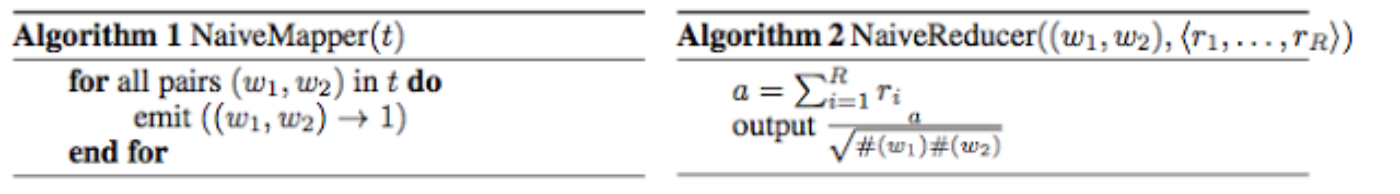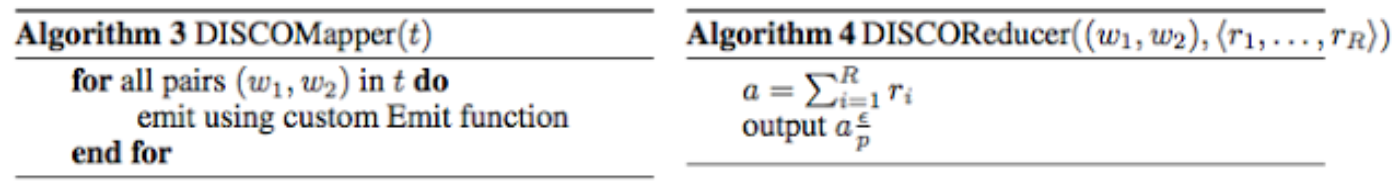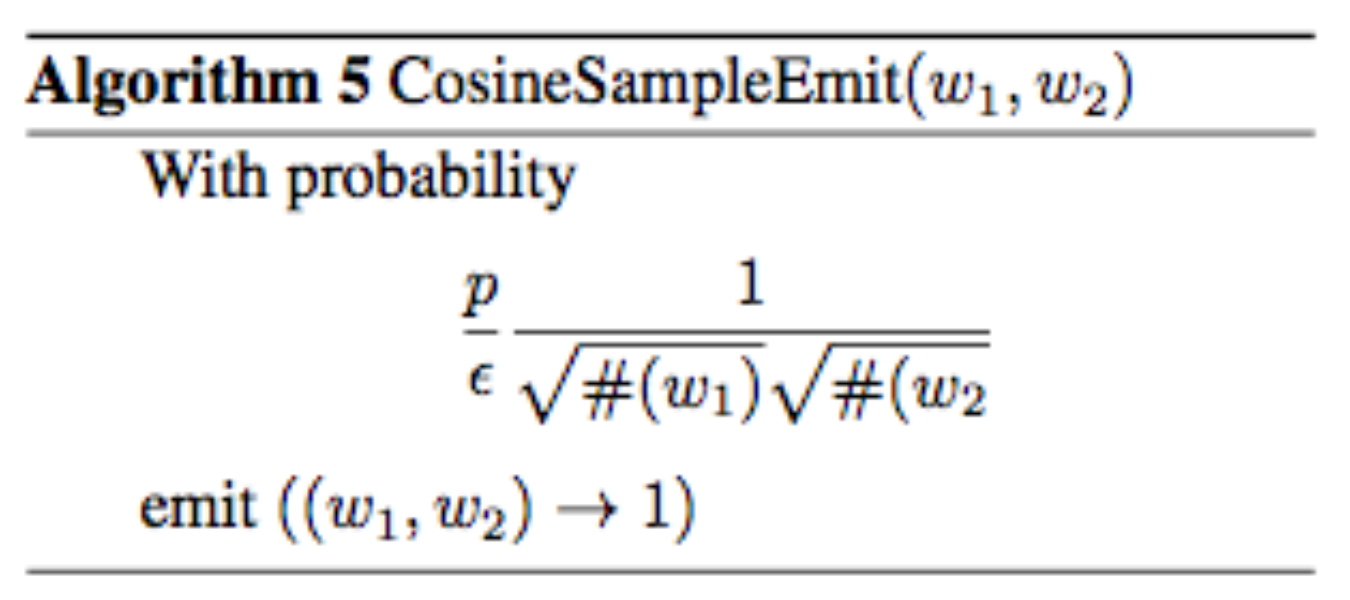# Dimension Independent Similarity Computation (DISCO)

By
Monday, 12 November 2012

MapReduce is a programming model for processing large data sets, typically used to do distributed computing on clusters of commodity computers. With large amount of processing power at hand, it’s very tempting to solve problems by brute force. However, we often combine clever sampling techniques with the power of MapReduce to extend its utility.

Consider the problem of finding all pairs of similarities between D indicator (0/1 entries) vectors, each of dimension N. In particular we focus on cosine similarities between all pairs of D vectors in R^N. Further assume that each dimension is L-sparse, meaning each dimension has at most L non-zeros across all points. For example, typical values to compute similarities between all pairs of a subset of Twitter users can be:

D = 10M
N = 1B
L = 1000

Since the dimensions are sparse, it is natural to store the points dimension by dimension. To compute cosine similarities, we can easily feed each dimension t into MapReduce by using the following Mapper and Reducer combinationWhere #(w) counts the number of dimensions in which point w occurs, and #(w1, w2) counts the number of dimensions in which w1 and w2 co-occur, i.e., the dot product between w1 and w2. The steps above compute all dot products, which will then be scaled by the cosine normalization factor.

There are two main complexity measures for MapReduce: “shuffle size”, and “reduce-key complexity”, defined shortly (Ashish Goel and Kamesh Munagala 2012). It can be easily shown that the above mappers will output on the order of O(NL^2) emissions, which for the example parameters we gave is infeasible. The number of emissions in the map phase is called the “shuffle size”, since that data needs to be shuffled around the network to reach the correct reducer.

Furthermore, the maximum number of items reduced to a single key is at most #(w1, w2), which can be as large as N. Thus the “reduce-key complexity” for the above scheme is N.

We can drastically reduce the shuffle size and reduce-key complexity by some clever sampling:Notation: p and ε are oversampling parameters.

In this case, the output of the reducers are random variables whose expectations are the cosine similarities. Two proofs are needed to justify the effectiveness of this scheme. First, that the expectations are indeed correct and obtained with high probability, and second, that the shuffle size is greatly reduced.

We prove both of these claims in (Reza Bosagh-Zadeh and Ashish Goel 2012). In particular, in addition to correctness, we prove that the shuffle size of the above scheme is only O(DL log(D)/ε), with no dependence on the “dimension” N, hence the name.

This means as long as you have enough mappers to read your data, you can use the DISCO sampling scheme to make the shuffle size tractable. Furthermore, each reduce key gets at most O(log(D)/ε) values, thus making the reduce-key complexity tractable too.

Within Twitter, we use the DISCO sampling scheme to compute similar users. We have also used the scheme to find highly similar pairs of words, by taking each dimension to be the indicator vector that signals in which Tweets the word appears. We further empirically verify the claims and observe large reductions in shuffle size, with details in the paper.

Finally, this sampling scheme can be used to implement many other similarity measures. For Jaccard Similarity, we improve the implementation of the well-known MinHash (http://en.wikipedia.org/wiki/MinHash) scheme on Map-Reduce.

Posted by
Reza Zadeh (@Reza_Zadeh) and Ashish Goel (@ashishgoel) - Personalization & Recommendation Systems Group and Revenue Group

Bosagh-Zadeh, Reza and Goel, Ashish (2012), Dimension Independent Similarity Computation, arXiv:1206.2082

Goel, Ashish and Munagala, Kamesh (2012), Complexity Measures for Map-Reduce, and Comparison to Parallel Computing, http://www.stanford.edu/~ashishg/papers/mapreducecomplexity.pdf